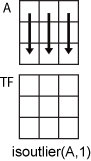isoutlier

说明

TF = isoutlier(A) 返回一个逻辑数组，当在 A 的元素中检测到离群值时，该数组中与之对应的元素为 true。默认情况下，离群值是指与中位数相差超过三倍经过换算的中位数绝对偏差 (MAD) 的值。如果 A 是矩阵或表，则 isoutlier 分别对每一列进行运算。如果 A 是多维数组，则 isoutlier 沿大小不等于 1 的第一个维度进行运算。

TF = isoutlier(A,method) 指定检测离群值的方法。例如，isoutlier(A,'mean') 对偏离均值超过三倍标准差的所有元素返回 true

TF = isoutlier(A,'percentiles',threshold) 将离群值定义为 threshold 所指定的百分位数以外的点。threshold 参数是包含上下百分位数阈值的二元素行向量，例如 [10 90]

TF = isoutlier(A,movmethod,window) 指定根据 window 定义的窗口长度检测局部离群值的移动方法。例如，isoutlier(A,'movmedian',5) 对包含五个元素的滑动窗内偏离局部中位数超过三倍局部换算 MAD 的所有元素返回 true

TF = isoutlier(___,Name,Value) 使用一个或多个名称-值对组参数指定用于检测离群值的其他参数。例如，isoutlier(A,'SamplePoints',t) 相对于时间向量 t 中的对应元素检测 A 中的离群值。

[TF,L,U,C] = isoutlier(___) 还返回离群值检测方法使用的下阈值、上阈值和中心值。

示例

A = [57 59 60 100 59 58 57 58 300 61 62 60 62 58 57];
TF = isoutlier(A)
TF = 1x15 logical array

0   0   0   1   0   0   0   0   1   0   0   0   0   0   0

A = [57 59 60 100 59 58 57 58 300 61 62 60 62 58 57];
TF = isoutlier(A,'mean')
TF = 1x15 logical array

0   0   0   0   0   0   0   0   1   0   0   0   0   0   0

x = -2*pi:0.1:2*pi;
A = sin(x);
A(47) = 0;

t = datetime(2017,1,1,0,0,0) + hours(0:length(x)-1);

TF = isoutlier(A,'movmedian',hours(5),'SamplePoints',t);
plot(t,A,t(TF),A(TF),'x')
legend('Data','Outlier')A = magic(5) + diag(200*ones(1,5))
A = 5×5

217    24     1     8    15
23   205     7    14    16
4     6   213    20    22
10    12    19   221     3
11    18    25     2   209

TF = isoutlier(A,2)
TF = 5x5 logical array

1   0   0   0   0
0   1   0   0   0
0   0   1   0   0
0   0   0   1   0
0   0   0   0   1

x = 1:10;
A = [60 59 49 49 58 100 61 57 48 58];
[TF,L,U,C] = isoutlier(A);
plot(x,A,x(TF),A(TF),'x',x,L*ones(1,10),x,U*ones(1,10),x,C*ones(1,10))
legend('Original Data','Outlier','Lower Threshold','Upper Threshold','Center Value')输入参数

'mean'对偏离均值超过三倍标准差的元素返回 true。此方法比 'median' 快，但没有它可靠。
'quartiles'对高于上四分位数或低于下四分位数超过 1.5 个四分位范围的元素返回 true。当 A 中的数据不是正态分布时，此方法很有用。
'grubbs'应用 Grubbs 检验以检测离群值，即基于假设检验每次迭代删除一个离群值。此方法假设 A 中的数据呈正态分布。
'gesd'应用广义极端 Student 化偏差检验检测离群值。此迭代方法与 'grubbs' 类似，但当有多个离群值互相遮盖时，此方法的执行效果更好。

'movmedian'对在 window 指定的窗口长度内偏离局部中位数超过三倍局部换算 MAD 的元素返回 true。此方法也称为 Hampel 滤波器
'movmean'对在 window 指定的窗口长度内偏离局部中值超过三个局部标准差的元素返回 true

A 是时间表或 'SamplePoints' 指定为 datetimeduration 向量时，window 的类型必须为 duration，并会相对于样本点来计算窗口。

isoutlier(A,1) 基于 A 中每一列的数据检测离群值。isoutlier(A,2) 基于 A 中每一行的数据检测离群值。A 为表或时间表时，不支持 dimisoutlier 分别对每个表或时间表变量进行运算。

名称-值对组参数

'Var1'

"Var1"

{'Var1' 'Var2'}

["Var1" "Var2"]

1

[1 3 5]

[true false true]

@isnumeric

vartype 下标

vartype 函数生成的表下标

vartype('numeric')

'MaxNumOutliers' 的默认值是最接近 A 中的元素数的 10% 的整数。为离群值数上限设置较大的值可以确保检测到所有离群值，但代价是降低计算效率。

'gesd' 方法假定非离群值输入数据是从近似正态分布采样的。如果数据不是以这种方式采样的，则返回的离群值数量可能会超过 'MaxNumOutliers' 值。

详细信息

中位数绝对偏差

，其中 i = 1,2,...,N。